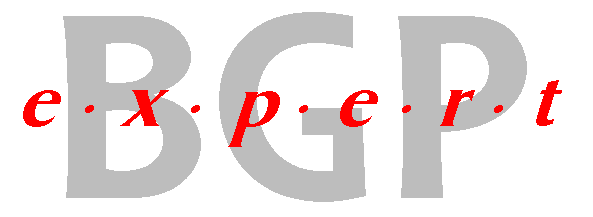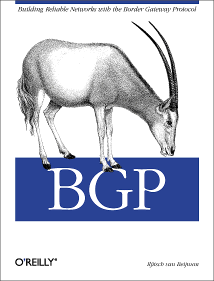(advertisement)
 Counting 32-bit AS numbers (posted 2011-03-17) I've had a page that shows how many autonomous system numbers the RIRs have given out for a while now. However, when updating the slides for monday's BGP training course I realized that the results are all AS numbers—regardless of whether they're 16- or 32-bit. So I updated the page. You can now request either 16-bit AS numbers, 32-bit AS numbers, or both. The total number of AS numbers given out so far is 53780. 1744 of those are numbers above 65535, so they're 32-bit. I was actually surprised that the number is this high. So far this year, the RIPE NCC has given out 592 AS numbers (that's more than half of the world total!), 199 of which are 32-bit. So it looks like 32-bit AS deployment is finally picking up. The number of 16-bit AS numbers given out is 52036, with some 4400 given out in both 2009 and 2010. So at this rate, the 16-bit AS number space will be exhausted in less than three years. Perform your own queries on the data here. An interesting one is the number of AS numbers per country. For instance, organizations in the US got 302 AS numbers this year so far. And only two of those are 32-bit.